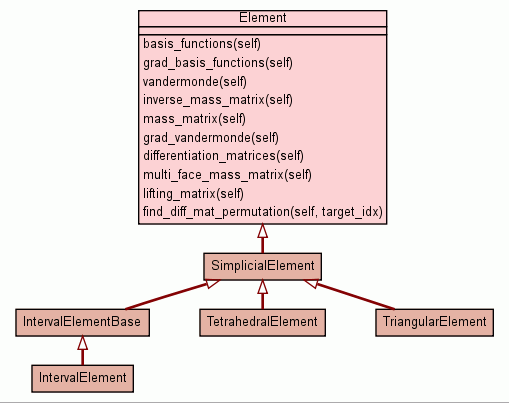[frames] | no frames]

# Class Element

source codeInstance Methods

 basis_functions(self) Get a sequence of functions that form a basis of the approximation space. source code

 grad_basis_functions(self) Get the gradient functions of the basis_functions(), in the same order. source code

 vandermonde(self) source code

 inverse_mass_matrix(self) Return the inverse of the mass matrix of the unit element with respect to the nodal coefficients. source code

 mass_matrix(self) Return the mass matrix of the unit element with respect to the nodal coefficients. source code

 differentiation_matrices(self) Return matrices that map the nodal values of a function to the nodal values of its derivative in each of the unit coordinate directions. source code

 multi_face_mass_matrix(self) Return a matrix that combines the effect of multiple face mass matrices applied to a vector of the shape: source code

 lifting_matrix(self) Return a matrix that combines the effect of the inverse mass matrix applied after the multi-face mass matrix to a vector of the shape: source code

 find_diff_mat_permutation(self, target_idx) Find a permuation `p` such that: source code

Inherited from `object`: `__delattr__`, `__getattribute__`, `__hash__`, `__init__`, `__new__`, `__reduce__`, `__reduce_ex__`, `__repr__`, `__setattr__`, `__str__`

 Properties

Inherited from `object`: `__class__`

 Method Details

### vandermonde(self)

source code
Decorators:
• `@memoize_method`

### inverse_mass_matrix(self)

source code

Return the inverse of the mass matrix of the unit element with respect to the nodal coefficients. Divide by the Jacobian to obtain the global mass matrix.

Decorators:
• `@memoize_method`

### mass_matrix(self)

source code

Return the mass matrix of the unit element with respect to the nodal coefficients. Multiply by the Jacobian to obtain the global mass matrix.

Decorators:
• `@memoize_method`

source code

Compute the Vandermonde matrices of the grad_basis_functions(). Return a list of these matrices.

Decorators:
• `@memoize_method`

### differentiation_matrices(self)

source code

Return matrices that map the nodal values of a function to the nodal values of its derivative in each of the unit coordinate directions.

Decorators:
• `@memoize_method`

### multi_face_mass_matrix(self)

source code

Return a matrix that combines the effect of multiple face mass matrices applied to a vector of the shape:

```   [face_1_dofs]+[face_2_dofs]+...+[face_n_dofs]
```

Observe that this automatically maps this vector to a volume contribution.

Decorators:
• `@memoize_method`

### lifting_matrix(self)

source code

Return a matrix that combines the effect of the inverse mass matrix applied after the multi-face mass matrix to a vector of the shape:

```   [face_1_dofs]+[face_2_dofs]+...+[face_n_dofs]
```

Observe that this automatically maps this vector to a volume contribution.

Decorators:
• `@memoize_method`

### find_diff_mat_permutation(self, target_idx)

source code

Find a permuation `p` such that:

```   diff_mats = self.differentiation_matrices()
diff_mats[p][:,p] == diff_mats[target_idx]
```

The permutation is returned as a numpy array of type intp.

 Generated by Epydoc 3.0.1 on Sat Aug 29 14:33:24 2009 http://epydoc.sourceforge.net